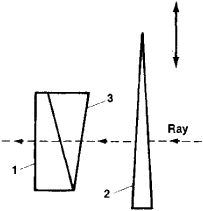# Saccharimeter

Also found in: Dictionary, Medical, Wikipedia.
Related to Saccharimeter: saccharometer, polarimeter, manometer, lactometer, ammeter

## saccharimeter

[‚sak·ə′rim·əd·ər]
(engineering)
An instrument for measuring the amount of sugar in a solution, often by determining the change in polarization produced by the solution.

## Saccharimeter

a polarization device that determines the sugar content of solutions by measuring the angle of rotation of the plane of polarization of a light beam. The amount of rotation is proportional to the concentration of the solution. Sac-charimeters are used less frequently for measuring concentrations of other optically active substances.Figure 1. Quartz compensator of a saccharimeter: (1) stationary wedge of dextrorotatory quartz, (2) movable wedge of levorotatory quartz, and (3) auxiliary wedge of glass. The movable quartz wedge is connected to a scale and slides in the directions indicated by the vertical arrow. A zero reading on the scale corresponds to the position of the movable wedge at which the effects of the two quartz wedges are compensated. The glass auxiliary wedge is introduced to prevent a change in direction of the light ray passing through the quartz wedges.

Rotation in a saccharimeter is compensated, that is, the plane of polarization is rotated by an amount equal in magnitude and opposite in direction to the rotation produced by the solution. The compensator in a saccharimeter is a linearly movable quartz wedge (Figure 1) rather than the rotating analyzer of a polarimeter. The dependence of optical activity on the wavelength of the light, that is, the rotatory dispersion, of quartz and sugar are nearly identical. This circumstance allows the solution to be illuminated by white light when a quartz compensator is used in determining the concentration of sugar. The scale along which the compensating wedge travels is calibrated to give a direct reading of the percentage concentration of optically active substance in the solution. Compensation with sac-charimeters, as with polarimeters, involves an equalization of brightnesses of two halves of the field of view. Conditions for measuring sugar content with a saccharimeter have been standardized: the incident white light must first be filtered through a 1.5-cm layer of 6-percent potassium dichromate (K2Cr2O7) solution.

In many modern saccharimeters with polarizational modulation of light, the quartz compensator and scale are connected to a tracking system, and the rotation plane of polarization is compensated automatically.

### REFERENCES

Landsberg, G. S. Optika, 4th ed. (Obshchii kurs fiziki, vol. 3.) Moscow, 1957.
Shishlovskii, A. A. Prikladnaia fizicheskaia optika. Moscow, 1961.
Silin, P. M., and N. P. Silina. Khimicheskii kontrol’ sveklosakharnogo proizvodstva, 3rd ed. Moscow, 1960.
Site: Follow: Share:
Open / Close